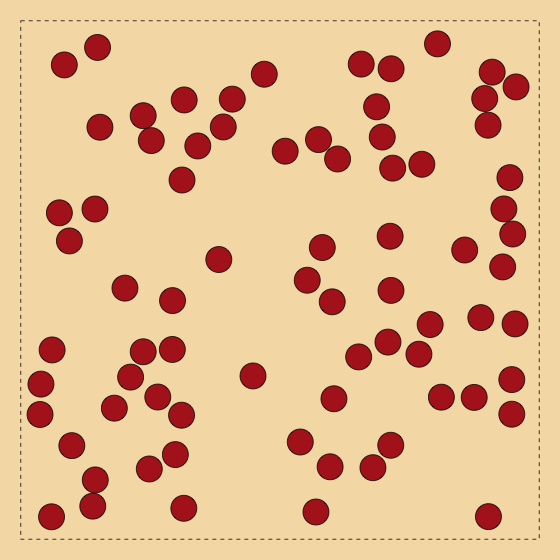# Establishing the Idea

## Why Geocomputation ?

• … and not
• GI Science
• Geography
• Spatial Statistics
• … for example?

## Need more than ‘standard’ GIS:

“GIS was, for some, a backwards step because the data models and analysis methods provided were simply not rich enough in geographical concepts and understanding to meet their needs.”

http://www.geocomputation.org/what.html

Needs such as:

• Fitting new (but more appropriate) models
• Searching for spatial pattern
• Visualisation
• Knowledge discovery
• Exploratory data analysis?

# Fitting new models

## Its not just about the models - its also about the approaches

‘Classical’ spatial statistics:
Assume $$y_i = X\beta + \epsilon$$ where $$\epsilon = W\epsilon + \nu$$ and $$\nu_i \sim N(0,\sigma^2)$$
• But why? Were does this model come from?
• Why is it linear?
• Why does it depend on a specific set of spatial units? What about the MAUP?

“This lack of a well-defined link between process and form is commonplace in spatial analysis, and is well-documented in fields such as point set clustering and fractal analysis. That it also applies here, in spatial regression modeling, should come as no surprise.” De Smith, Goodchild, Longley 2007 - Geospatial Analysis: A Comprehensive Guide to Principles, Techniques and Software Tools P243

## Process-oriented approaches

• Some excellent ones exist
• Cellular automata
• Agent-based models
• Microsimulation
• All more grounded in reality
• But some issues not fully addressed
• Calibration
• Model selection
• Hypothesis testing
• Maybe these are done better by classical approaches

## A geocomputation solution?

• Approximate Bayesian Computation (ABC)
• See eg Marjoram, P., J. Molitor, V. Plagnol and S. Tavaré. Markov chain Monte Carlo without likelihoods. Proceedings of the National Academy of Sciences USA 100: 15324–15328.
• Simplifying massively:
• this allows you to make Bayesian inferences about processes you can simulate
• even if the likelihood is intractable

## A Quick overview

• Draw parameter values from a prior distribution
• Use these for the simulation
• Keep them in a set of successful parameters if sufficiently ‘near’ to the real data
• repeat these steps LOTS of times
• the successful parameters have a distribution that should approximate the Bayesian posterior
• Throw mud at the wall and see what sticks

## Example - 2D hardcore point process• Random points but
• Always separated by a distance $$d$$
• Models
• Locations of coins on fairground game
• Locations of settlements?
• Locations of animal nests?
• Easy to simulate
• Hard to manage analytically
• How to estimate $$d$$?